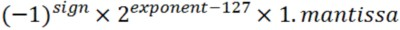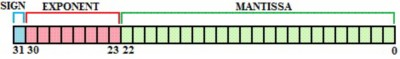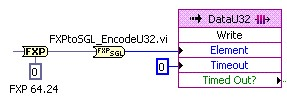# Archived: Fixed-Point (FXP) to Single (SGL) Conversion: LabVIEW 2011 FPGA Module and Earlier

NI does not actively maintain this document.

This content provides support for older products and technology, so you may notice outdated links or obsolete information about operating systems or other relevant products.

## Visão geral

Beginning with the LabVIEW 2012 FPGA Module, you can perform fixed-point to floating point conversions using the To Single Precision Float function. The following applies to the LabVIEW 2011 FPGA Module and earlier.

The fixed-point data type used by LabVIEW FPGA is a useful feature especially when working with NI C Series modules. In many applications it is necessary to convert fixed-point data to a floating-point representation for processing or streaming operations.

The conversion from fixed-point to floating point may certainly be done by the windows or real-time host application, but depending on available computing resources it can be advantageous to perform that conversion down on the FPGA.

LabVIEW FPGA does not natively support floating-point data types, but an understanding of IEEE Std 754-2008 standard (for floating point binary representation) will enable us to encode floating-point data types as unsigned integers.   For example single-precision floating-point data can be stored as an unsigned 32 bit word (U32) on the FPGA and then typecast into a SGL precision floating-point value by the host application.  A typecast from U32 to SGL is much cheaper (in terms of CPU resources) than a conversion from FXP to SGL.

## Single Precision Binary Floating-Point Format

IEEE 754 standard defines this data type as:• Sign: 1 bit
• Exponent width: 8 bits
• Significant precision: 23 bits (24 implicit)The mantissa assumes the most significant bit is 1 so only 23 bits of the fractional mantissa is actually encoded.  With a 24-bit mantissa,  you will get approximately 6 1/2 decimals digits of precision or >120 dB of dynamic range.

The exponent value is is biased by 127 to make floating point conversions more efficient.

## Conversion from Decimal to Exponent / Mantissa Form

To represent a decimal number in the exponent / mantissa form you must:

1. Divide the number by 2^(most significant bit)
2. The precision digits of the remaining value becomes the mantissa.
3. The position of the most significant bit (biased by 127) becomes the exponent

Example:
To convert 9.5 from a decimal value to the exponent / mantissa form:

1. Divide 9.5 by 8
2. The remainder is 1.1875 so the mantissa is 0.1875
3. The exponent is 130 (position 3 + 127)

## Conversion from Fixed-Point to SGL Precision Floating-Point

A 64.24 fixed-point value reserves 24 bits for the integer and 40 bits for the precision digits.  The value 9.5 would be represented as:

40 bits of precision >>    <<  24 integer bits

00000000 00000000 00000000 00000000 00000001 .  1001000 00000000 00000000

To perform the conversion in binary, the algorithm is much simpler:

1. Find the position of the MSB and bias it by 127 to get the exponent
2. Extract the 23 bits behind the MSB and use that for the mantissa

## Implementing the Conversion on FPGA

While the algorithm mentioned above is simply stated, the most difficult part of the data conversion is finding the position of the most significant bit.

Ultimately, a searching algorithm must be used.  The LV FPGA conversion code provided here combines a single-cycle Timed Loop (SCTL) and a state machine architecture to perform the search and encode the data in the least amount of time.  The state machine execution follows this progression:

1. Initialize (1 iteration):  In this state we typecast the 64 bit FXP data into a U64 integer which will be searched in the upcoming state.  The initialize state also checks to see if the sign bit will be 1 or 0.
2. Search (6 iterations for a 64 bit word):  In this state we split the incoming word into a Hi and Lo array. If the Hi array is greater than or equal to 1 then we set a MSB search register to the first index of the Hi array and pass the Hi array to the next iteration to be split. Otherwise we pass the Lo array to the next iteration to be split. After 6 iterations the MSB register will equal the MSB of the 64 bit word.
3. Encode (1 iteration):  In this state we use the value of the MSB register to calculate the exponent value and to extract the 23 bit mantissa from the 64 bit word. Finally this state takes the 8 bit exponent, the 23 bit mantissa, and the 1 bit sign value and builds a U32 word according to the IEEE-754 standard for a single precision floating-point value.

## Execution Benchmarks

For each data point, the FXPtoSGL_EncodeU32.vi takes 10 ticks of the FPGA clock to execute.When compiled by itself on an NI cRIO-9074 the VI used:

• 1236 slices: 3% of FPGA fabric
• 2087 LUTs: 5% of FPGA fabric

## Software Requirements

The FXPtoSGL_EncodeU32.vi requires the following support software:

• LabVIEW 8.6
• LabVIEW FPGA 8.6